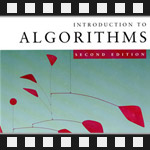This is the fourth post in an article series about MIT's lecture course "Introduction to Algorithms." In this post I will review lecture six, which is on the topic of Order Statistics.

The problem of order statistics can be described as following. Given a set of N elements, find k-th smallest element in it. For example, the minimum of a set is the first order statistic (k = 1) and the maximum is the N-th order statistic (k = N).

Lecture six addresses the problem of selecting the k-th order statistic from a set of N distinct numbers.

The lecture begins with discussing the naive algorithm for finding k-th order statistic -- sort the given set (or array) A of numbers and return k-th element A[k]. Running time of such algorithm at best is O(n·log(n)).

Erik Demaine (professor) presents a randomized divide and conquer algorithm for this problem (my second post covers divide and conquer algorithms), which runs in expected linear time.

The key idea of this algorithm is to call Randomized-Partition (from previous lecture) subroutine on the given array and then recursively conquer on the partitioned elements on the left and right of the chosen pivot element.

Here is the pseudo code of this algorithm:

```Randomized-Select(A, p, q, k) // finds k-th smallest element in array A[p..q]
if p == q
return A[p]
r = Randomized-Partition(A, p, q)
n = r - p + 1
if k == n
return A[r]
if k < n
return Randomized-Select(A, p, r-1, k)
else
return Randomized-Select(A, r+1, q, k-n)
```

The lecture continues with intuitive and then mathematically rigorous analysis of the algorithm. It is concluded that the expected running time of this algorithm is O(n) and the worst case (when the Randomized-Partition algorithm chooses bad pivots) is O(n2).

At the last minutes of the lecture a worst-case linear time order statistics algorithm is presented. This algorithm was invented by five scientists - M. Blum, R. W. Floyd, V. Pratt, R. Rivest and R. Tarjan. I found the original publication of this algorithm by these gentlemen: Time Bounds for Selection.

You're welcome to watch lecture six:

Lecture six notes:

Topics covered in lecture six:

• [00:30] The problem of order statistics.
• [01:50] Naive algorithm for finding k-th smallest element.
• [04:30] Randomized divide and conquer algorithm.
• [11:20] Example of algorithm run on array (6, 10, 13, 5, 8, 3, 2, 11) to find 7-th smallest element.
• [15:30] Intuitive analysis of Randomized-Select algorithm.
• [20:30] Mathematically rigorous analysis of expected running time.
• [43:55] Worst-case linear time order statistics algorithm.

Have fun! The next post will be all about hashing.